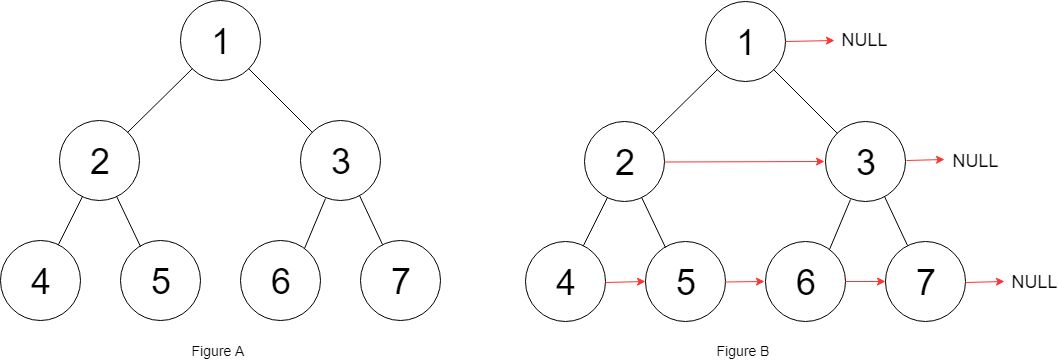# 116. Populating Next Right Pointers in Each Node

Medium
You are given a perfect binary tree where all leaves are on the same level, and every parent has two children. The binary tree has the following definition:
struct Node {
int val;
Node *left;
Node *right;
Node *next;
}
Populate each next pointer to point to its next right node. If there is no next right node, the next pointer should be set to `NULL`.
Initially, all next pointers are set to `NULL`.
Example 1:Input: root = [1,2,3,4,5,6,7]
Output:
[1,#,2,3,#,4,5,6,7,#]
Explanation:
Given the above perfect binary tree (Figure A), your function should populate each next pointer to point to its next right node, just like in Figure B. The serialized output is in level order as connected by the next pointers, with '#' signifying the end of each level.
Example 2:
Input: root = []
Output:
[]
Constraints:
• The number of nodes in the tree is in the range `[0, 212 - 1]`.
• `-1000 <= Node.val <= 1000`
Follow-up:
• You may only use constant extra space.
• The recursive approach is fine. You may assume implicit stack space does not count as extra space for this problem.

### 解題

Recursive
/**
* Definition for a Node.
* type Node struct {
* Val int
* Left *Node
* Right *Node
* Next *Node
* }
*/
func connect(root *Node) *Node {
if root == nil { return nil }
var helper func(*Node)
helper = func(root *Node) {
if root.Left != nil && root.Right != nil {
root.Left.Next = root.Right
if root.Next != nil {
root.Right.Next = root.Next.Left
}
helper(root.Left)
helper(root.Right)
}
return
}
helper(root)
return root
}
Queue
/**
* Definition for a Node.
* type Node struct {
* Val int
* Left *Node
* Right *Node
* Next *Node
* }
*/
func connect(root *Node) *Node {
if root == nil { return nil }
queue := []*Node{ root }
for len(queue) != 0 {
pop := queue
queue = queue[1:]
if pop.Left != nil && pop.Right != nil {
pop.Left.Next = pop.Right
if pop.Next != nil {
pop.Right.Next = pop.Next.Left
}
queue = append(queue, pop.Left)
queue = append(queue, pop.Right)
}
}
return root
}